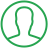# Fibonacci Series In Java Using Scanner

Last Updated : Jul 1, 2023In this article we will show you the solution of fibonacci series in java using scanner, in the Fibonacci series, the first two terms are 0 and 1, and the rest terms are formed by adding the two previous terms together.

Here are some explanations: 0, 1, 2, 3, 5, 8, and so forth.

A Java programme that prints the Fibonacci sequence up to n is required. When the programme is executed, the user must enter a value for n.

This program will print the first 5 terms of Fibonacci series if the value for n is 5.

These are the numbers from 0 to 1, 1, 2, 3, 5, 8, 13, 21 in the Fibonacci series in which the sum of previous terms equals the sum of the next two.

There are two terms in the Fibonacci sequence: 0 and 1.

As a mathematical expression, it can be written as follows:

The formula for Fn is Fn-1 + Fn-2

Where, Fn denotes the nth term of Fibonacci series.

The first two terms of this series are considered to be:

F0 = 0 (Zeroth term of Fibonacci sequence)

F1 = 1 (First term of Fibonacci sequence)

Users are asked to input Fibonacci series lengths in this Java program.

Getting the input is handled via Scanner class and its nextInt() function, and printing is handled via println().

We needed to import this package in our Java program since scanner class belongs to the java.util package.

## Step By Step Guide On Fibonacci Series In Java Using Scanner :-

```import java.util.Scanner;
class FibonacciProgram{
public static void main(String args[])
{
int n1=0,n2=1,n3,i,count=20;
System.out.print(n1+" "+n2);
for(i=4;i<count;++i)
{
n3=n1+n2;
System.out.print(" "+n3);
n1=n2;
n2=n3;
}
}}```
1. As the first step, we create an import function named java.util.Scanner*
2. Fibonacci Program is the class we create next.
3. As a string argument, we create a public static void main
4. Our next step is to create an integer number with n1, n2, n3 digits.
5. Our next step is to create the n1 and +n2 programs using system.out.println.
6. Then we create for loop for the program.
7. After that we again create the n1, n2 and n3.

## Conclusion :-

In order to call the functions of the Scanner class, we also needed to create an object of this class.

A series of numbers is generated by adding the last two numbers in the series using looping.

The first two elements are respectively started at 0 1 and 1.

I hope this article on fibonacci series in java using scanner helps you and the steps and method mentioned above are easy to follow and implement.

##About Pragati

Experienced coding content writer who enjoys breaking down complex concepts in programming languages like Java, Python, C, and C++. Over three years of experience producing interesting and relevant content for a variety of entities. Committed to providing concise and easy-to-understand articles that assist readers in easily understanding technology and industry trends in the world of coding and software development.